Скачать презентацию INVESTIGATING STUDENT DIFFICULTIES WITH CONCEPTS OF GRAVITATION Or

• Количество слайдов: 29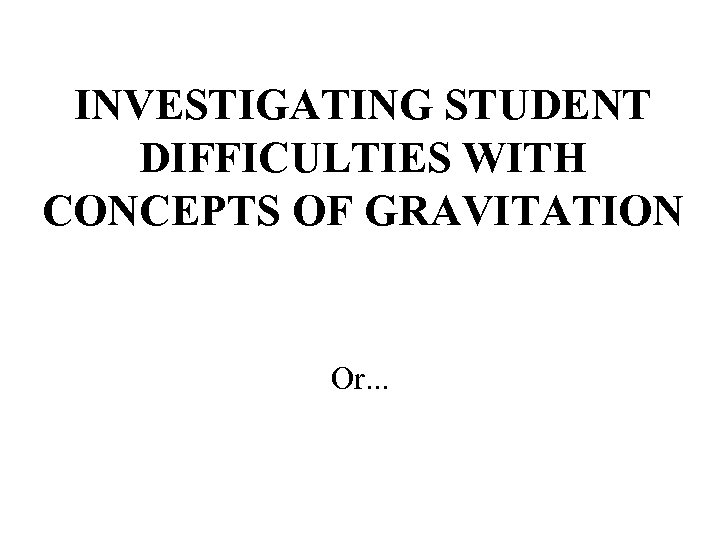INVESTIGATING STUDENT DIFFICULTIES WITH CONCEPTS OF GRAVITATION Or. . .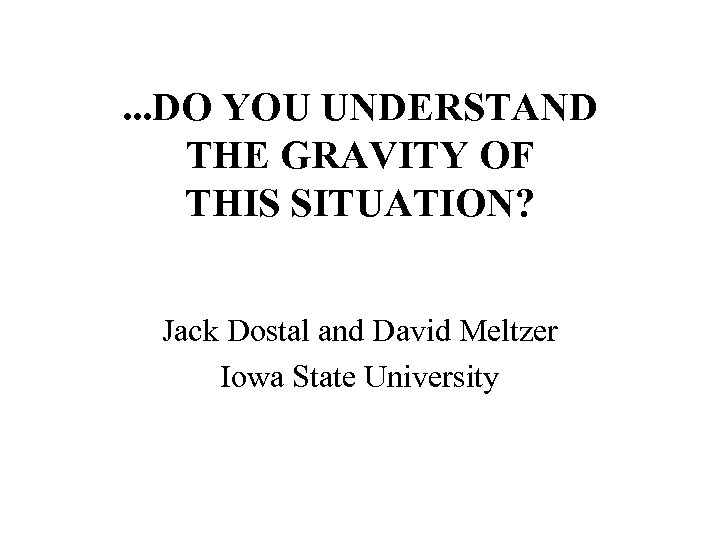. . . DO YOU UNDERSTAND THE GRAVITY OF THIS SITUATION? Jack Dostal and David Meltzer Iowa State University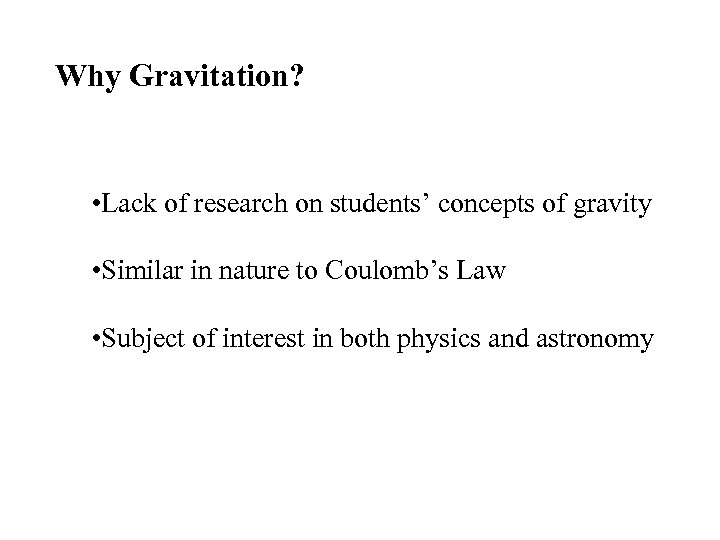Why Gravitation? • Lack of research on students’ concepts of gravity • Similar in nature to Coulomb’s Law • Subject of interest in both physics and astronomy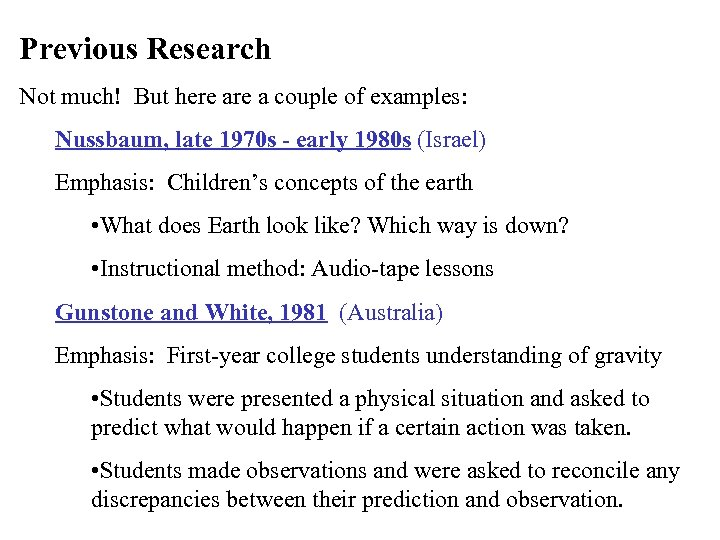Previous Research Not much! But here a couple of examples: Nussbaum, late 1970 s - early 1980 s (Israel) Emphasis: Children’s concepts of the earth • What does Earth look like? Which way is down? • Instructional method: Audio-tape lessons Gunstone and White, 1981 (Australia) Emphasis: First-year college students understanding of gravity • Students were presented a physical situation and asked to predict what would happen if a certain action was taken. • Students made observations and were asked to reconcile any discrepancies between their prediction and observation.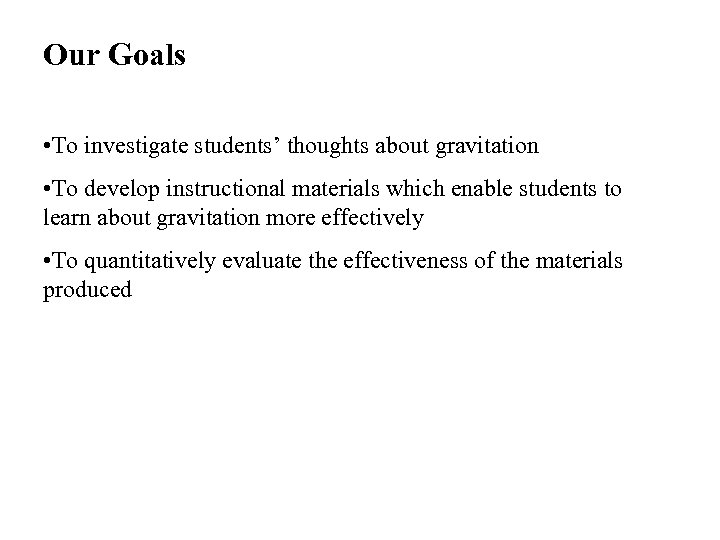Our Goals • To investigate students’ thoughts about gravitation • To develop instructional materials which enable students to learn about gravitation more effectively • To quantitatively evaluate the effectiveness of the materials produced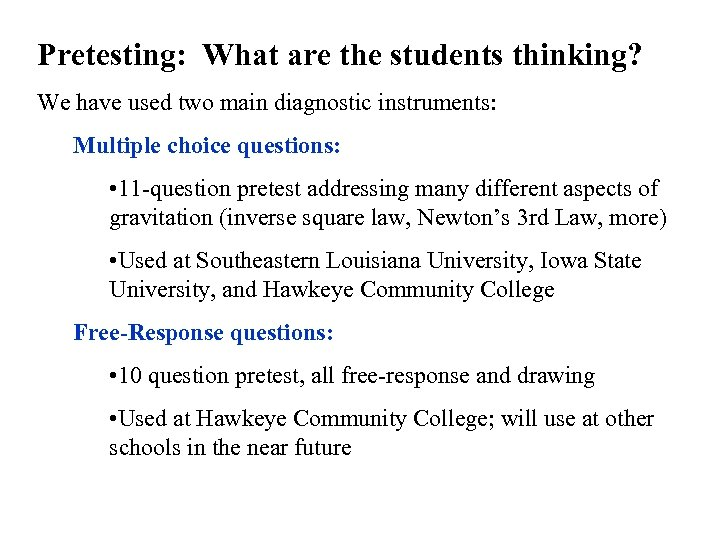Pretesting: What are the students thinking? We have used two main diagnostic instruments: Multiple choice questions: • 11 -question pretest addressing many different aspects of gravitation (inverse square law, Newton’s 3 rd Law, more) • Used at Southeastern Louisiana University, Iowa State University, and Hawkeye Community College Free-Response questions: • 10 question pretest, all free-response and drawing • Used at Hawkeye Community College; will use at other schools in the near future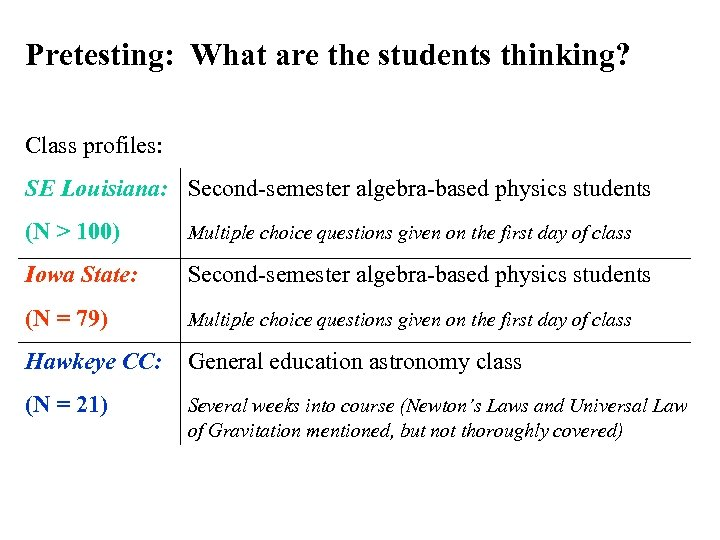Pretesting: What are the students thinking? Class profiles: SE Louisiana: Second-semester algebra-based physics students (N > 100) Multiple choice questions given on the first day of class Iowa State: Second-semester algebra-based physics students (N = 79) Multiple choice questions given on the first day of class Hawkeye CC: General education astronomy class (N = 21) Several weeks into course (Newton’s Laws and Universal Law of Gravitation mentioned, but not thoroughly covered)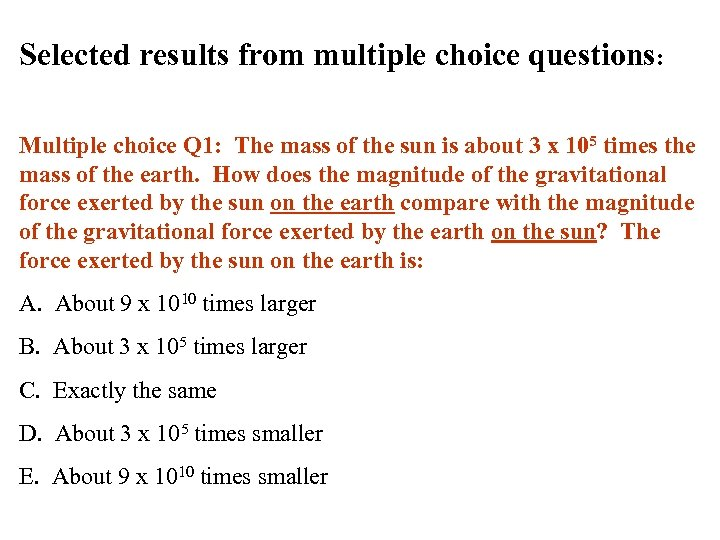Selected results from multiple choice questions: Multiple choice Q 1: The mass of the sun is about 3 x 105 times the mass of the earth. How does the magnitude of the gravitational force exerted by the sun on the earth compare with the magnitude of the gravitational force exerted by the earth on the sun? The force exerted by the sun on the earth is: A. About 9 x 1010 times larger B. About 3 x 105 times larger C. Exactly the same D. About 3 x 105 times smaller E. About 9 x 1010 times smaller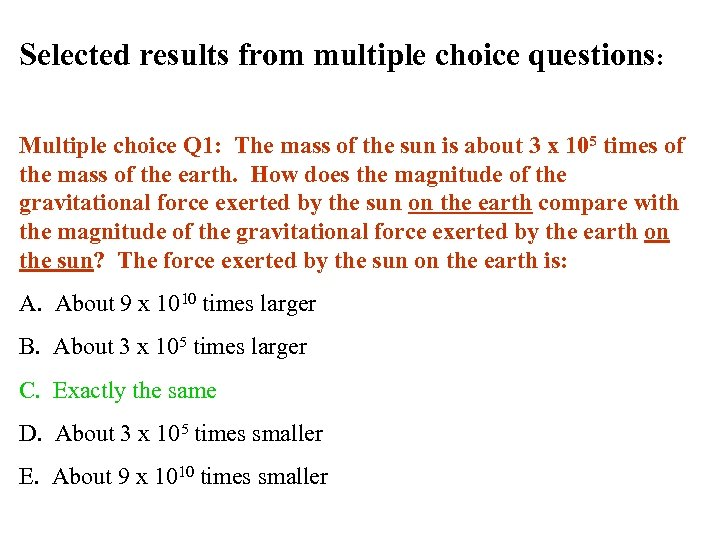Selected results from multiple choice questions: Multiple choice Q 1: The mass of the sun is about 3 x 105 times of the mass of the earth. How does the magnitude of the gravitational force exerted by the sun on the earth compare with the magnitude of the gravitational force exerted by the earth on the sun? The force exerted by the sun on the earth is: A. About 9 x 1010 times larger B. About 3 x 105 times larger C. Exactly the same D. About 3 x 105 times smaller E. About 9 x 1010 times smaller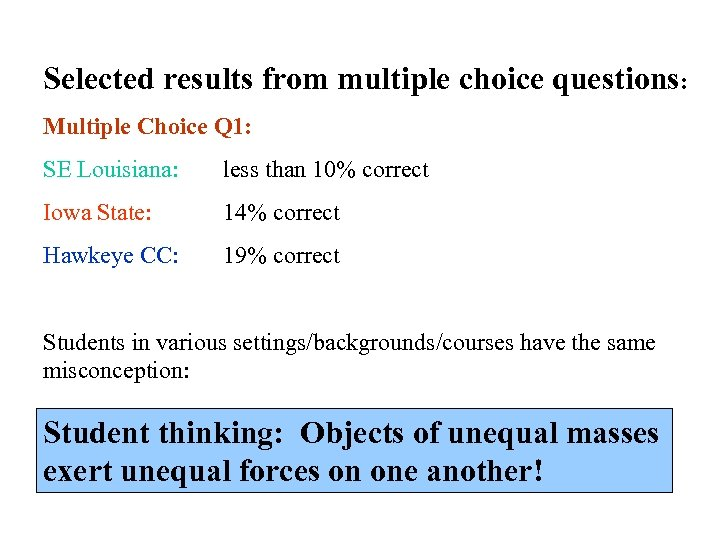Selected results from multiple choice questions: Multiple Choice Q 1: SE Louisiana: less than 10% correct Iowa State: 14% correct Hawkeye CC: 19% correct Students in various settings/backgrounds/courses have the same misconception: Student thinking: Objects of unequal masses exert unequal forces on one another!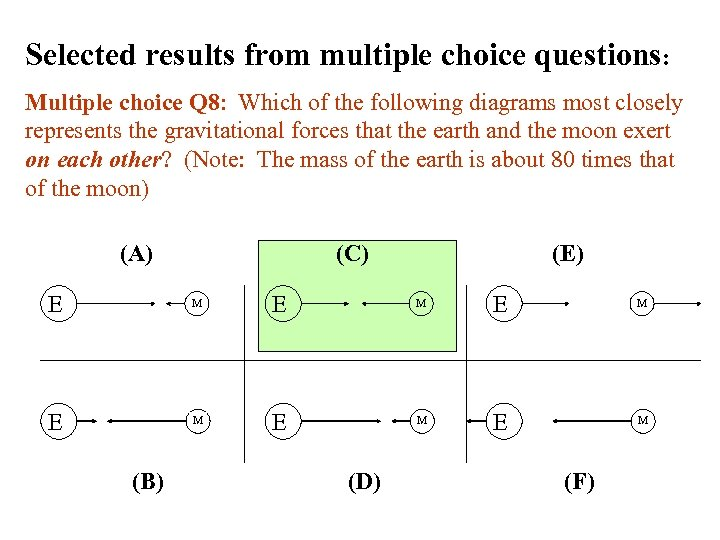Selected results from multiple choice questions: Multiple choice Q 8: Which of the following diagrams most closely represents the gravitational forces that the earth and the moon exert on each other? (Note: The mass of the earth is about 80 times that of the moon) (A) (C) (E) E M E M E M (B) (D) (F)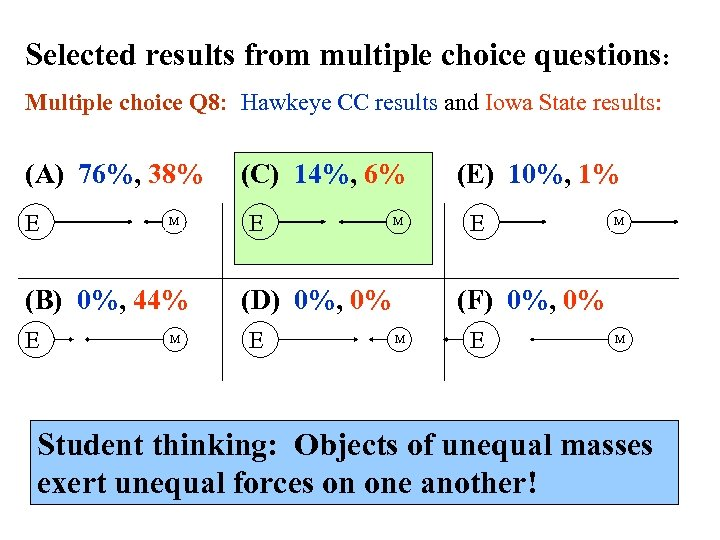Selected results from multiple choice questions: Multiple choice Q 8: Hawkeye CC results and Iowa State results: (A) 76%, 38% E M (B) 0%, 44% E M (C) 14%, 6% E M (D) 0%, 0% E (E) 10%, 1% E M (F) 0%, 0% M E M Student thinking: Objects of unequal masses exert unequal forces on one another!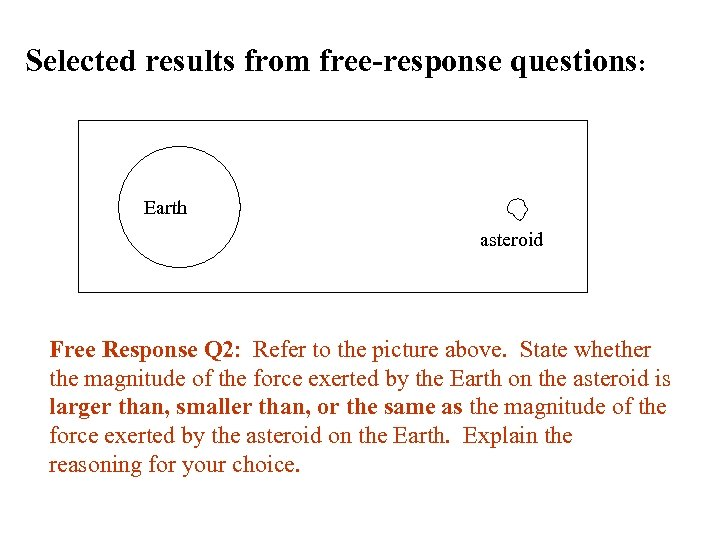Selected results from free-response questions: Earth asteroid Free Response Q 2: Refer to the picture above. State whether the magnitude of the force exerted by the Earth on the asteroid is larger than, smaller than, or the same as the magnitude of the force exerted by the asteroid on the Earth. Explain the reasoning for your choice.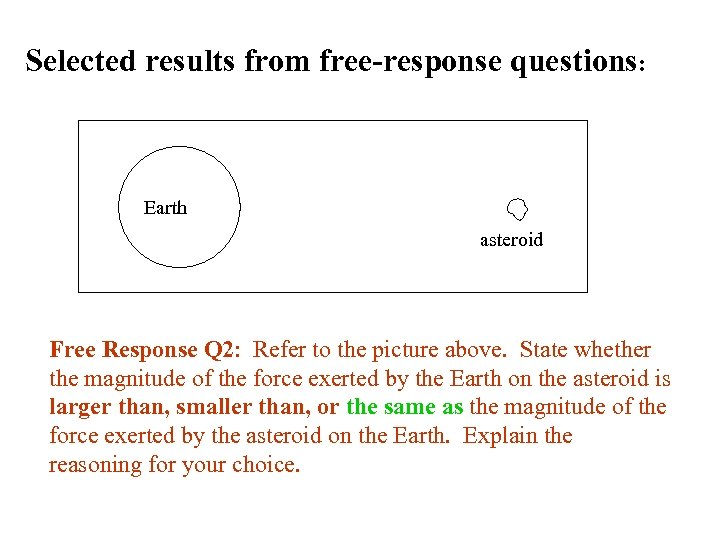Selected results from free-response questions: Earth asteroid Free Response Q 2: Refer to the picture above. State whether the magnitude of the force exerted by the Earth on the asteroid is larger than, smaller than, or the same as the magnitude of the force exerted by the asteroid on the Earth. Explain the reasoning for your choice.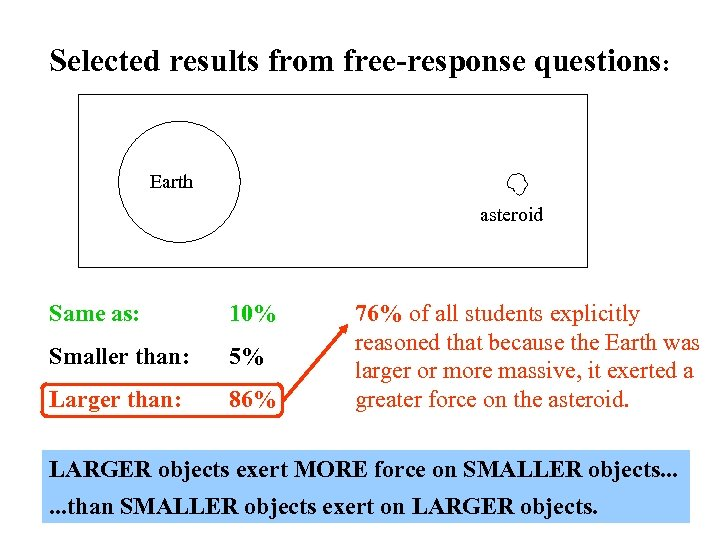Selected results from free-response questions: Earth asteroid Same as: 10% Smaller than: 5% Larger than: 86% 76% of all students explicitly reasoned that because the Earth was larger or more massive, it exerted a greater force on the asteroid. LARGER objects exert MORE force on SMALLER objects. . . than SMALLER objects exert on LARGER objects.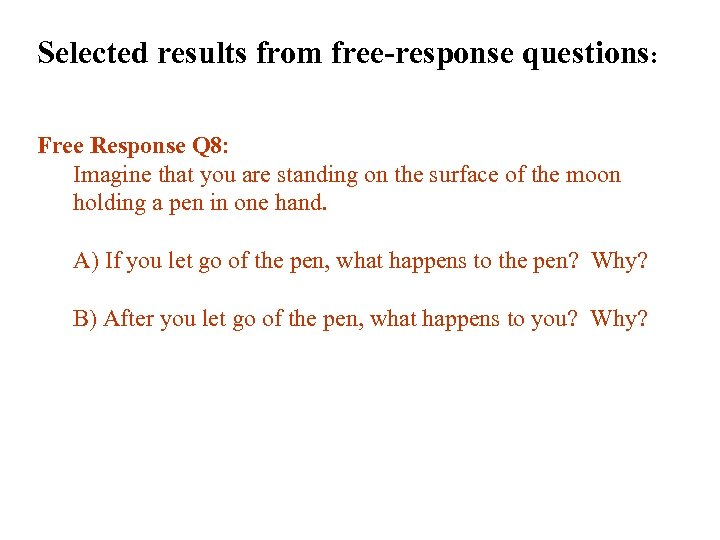Selected results from free-response questions: Free Response Q 8: Imagine that you are standing on the surface of the moon holding a pen in one hand. A) If you let go of the pen, what happens to the pen? Why? B) After you let go of the pen, what happens to you? Why?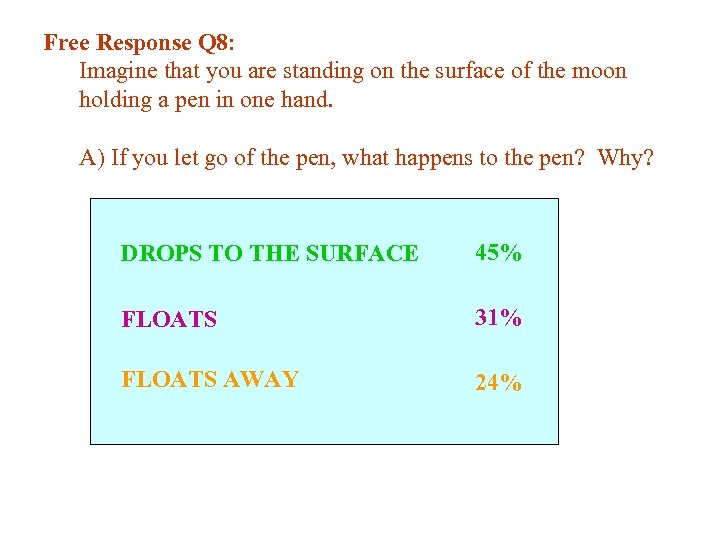Free Response Q 8: Imagine that you are standing on the surface of the moon holding a pen in one hand. A) If you let go of the pen, what happens to the pen? Why? DROPS TO THE SURFACE 45% FLOATS 31% FLOATS AWAY 24%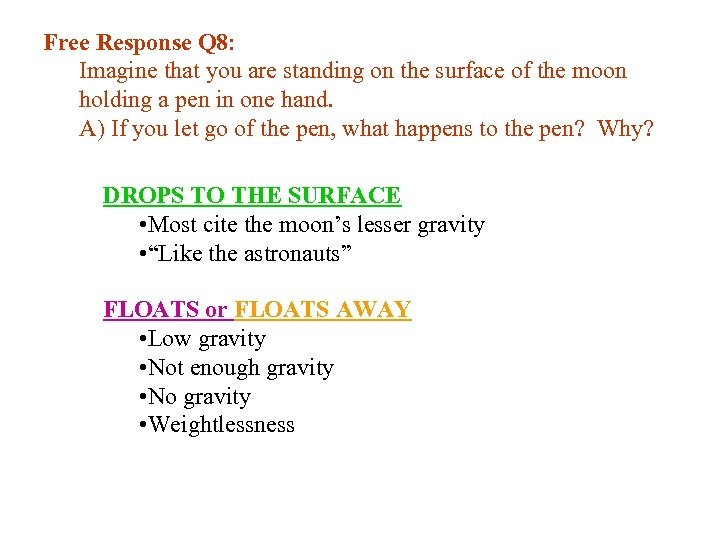Free Response Q 8: Imagine that you are standing on the surface of the moon holding a pen in one hand. A) If you let go of the pen, what happens to the pen? Why? DROPS TO THE SURFACE • Most cite the moon’s lesser gravity • “Like the astronauts” FLOATS or FLOATS AWAY • Low gravity • Not enough gravity • No gravity • Weightlessness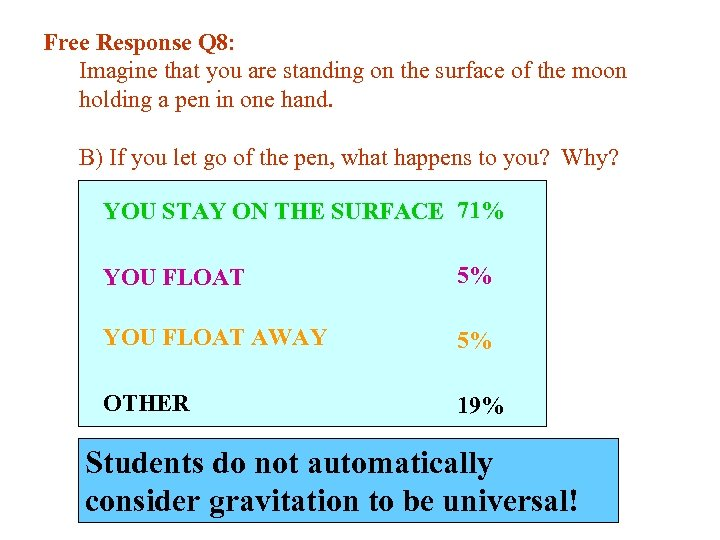Free Response Q 8: Imagine that you are standing on the surface of the moon holding a pen in one hand. B) If you let go of the pen, what happens to you? Why? YOU STAY ON THE SURFACE 71% YOU FLOAT 5% YOU FLOAT AWAY 5% OTHER 19% Students do not automatically consider gravitation to be universal!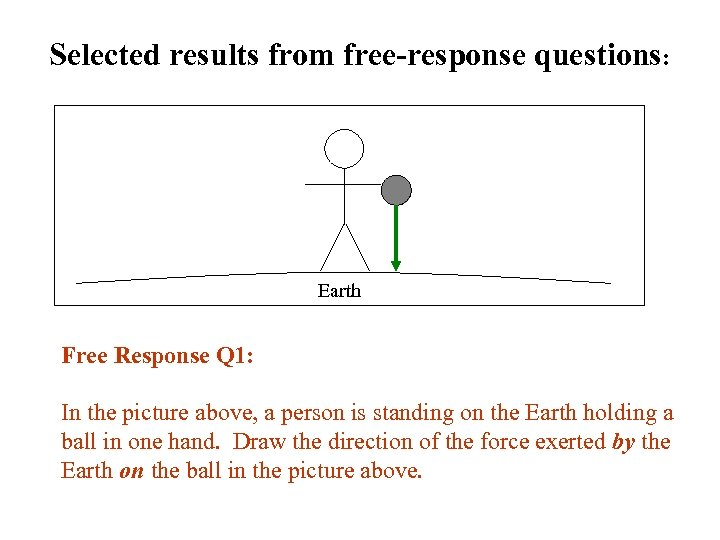Selected results from free-response questions: Earth Free Response Q 1: In the picture above, a person is standing on the Earth holding a ball in one hand. Draw the direction of the force exerted by the Earth on the ball in the picture above.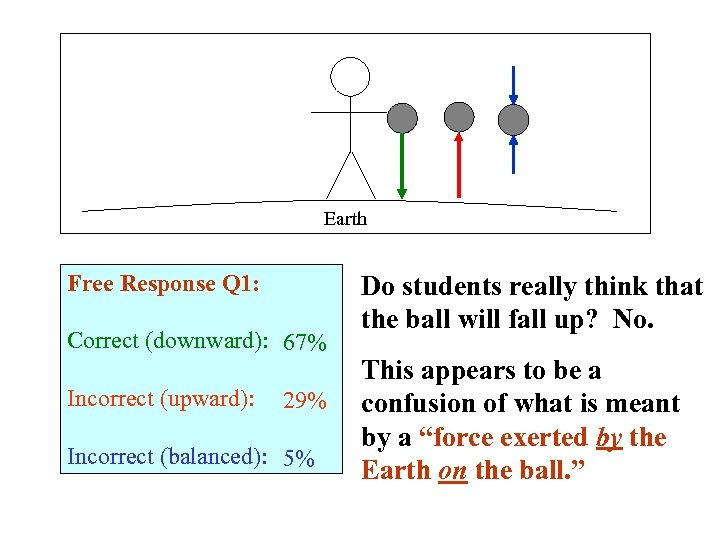Earth Free Response Q 1: Correct (downward): 67% Incorrect (upward): 29% Incorrect (balanced): 5% Do students really think that the ball will fall up? No. This appears to be a confusion of what is meant by a “force exerted by the Earth on the ball. ”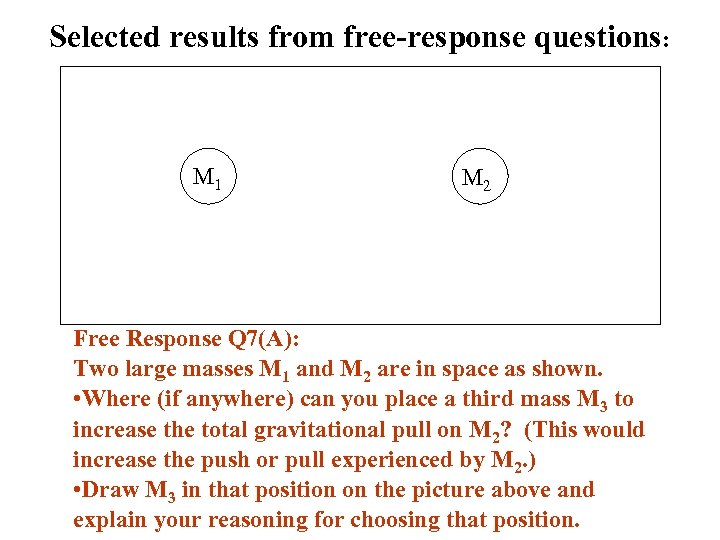Selected results from free-response questions: M 1 M 2 Free Response Q 7(A): Two large masses M 1 and M 2 are in space as shown. • Where (if anywhere) can you place a third mass M 3 to increase the total gravitational pull on M 2? (This would increase the push or pull experienced by M 2. ) • Draw M 3 in that position on the picture above and explain your reasoning for choosing that position.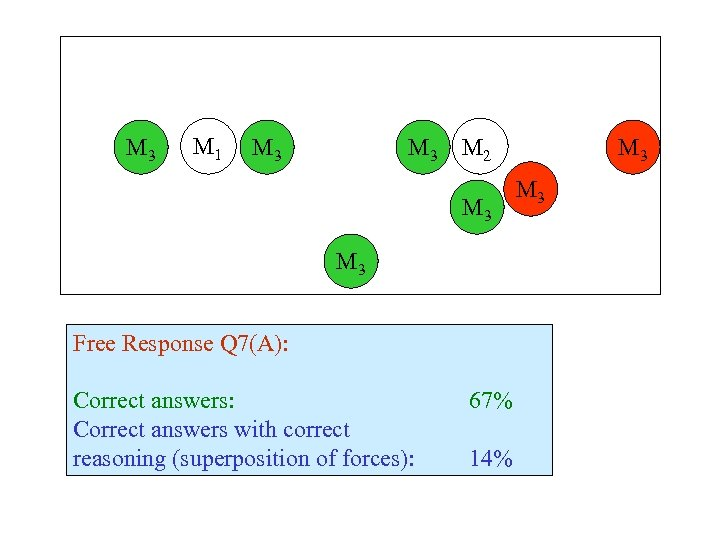M 3 M 1 M 3 M 2 M 3 Free Response Q 7(A): Correct answers with correct reasoning (superposition of forces): 67% 14% M 3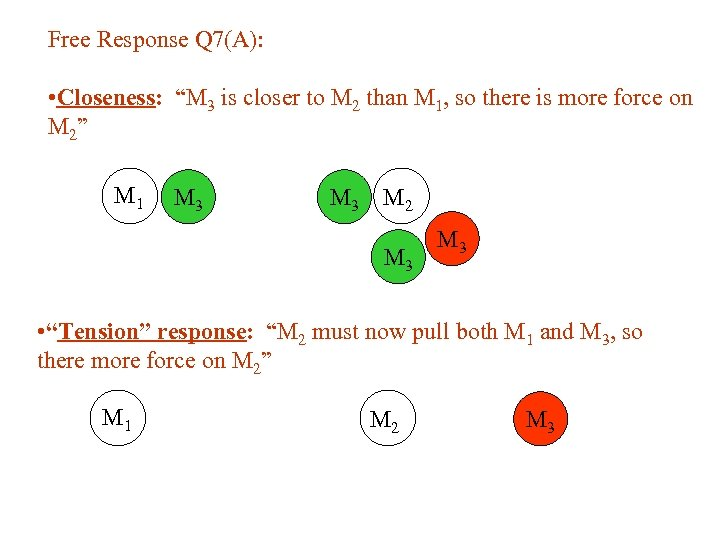Free Response Q 7(A): • Closeness: “M 3 is closer to M 2 than M 1, so there is more force on M 2” M 1 M 3 M 2 M 3 • “Tension” response: “M 2 must now pull both M 1 and M 3, so there more force on M 2” M 1 M 2 M 3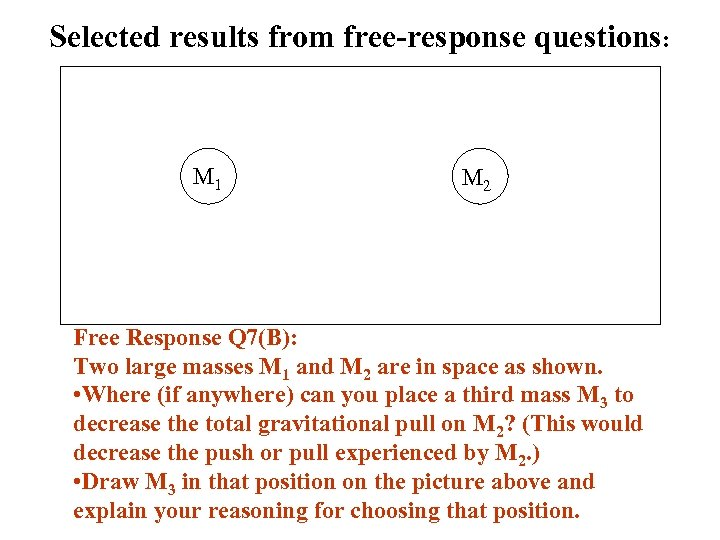Selected results from free-response questions: M 1 M 2 Free Response Q 7(B): Two large masses M 1 and M 2 are in space as shown. • Where (if anywhere) can you place a third mass M 3 to decrease the total gravitational pull on M 2? (This would decrease the push or pull experienced by M 2. ) • Draw M 3 in that position on the picture above and explain your reasoning for choosing that position.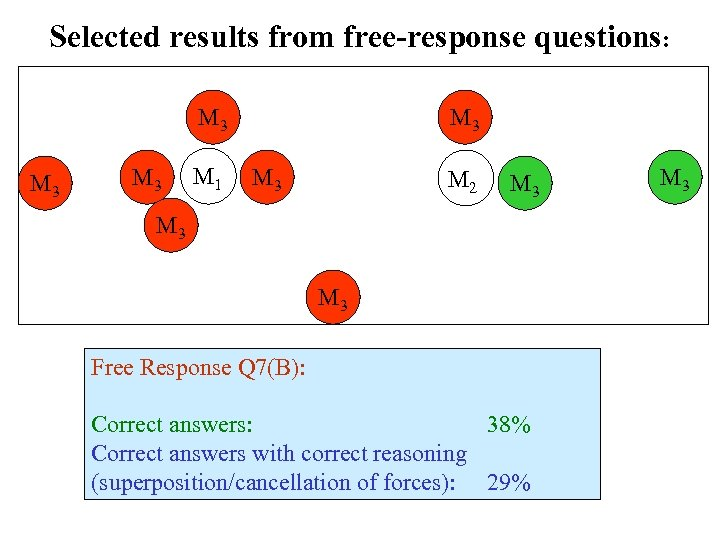Selected results from free-response questions: M 3 M 3 M 1 M 3 M 2 M 3 M 3 Free Response Q 7(B): Correct answers: 38% Correct answers with correct reasoning (superposition/cancellation of forces): 29% M 3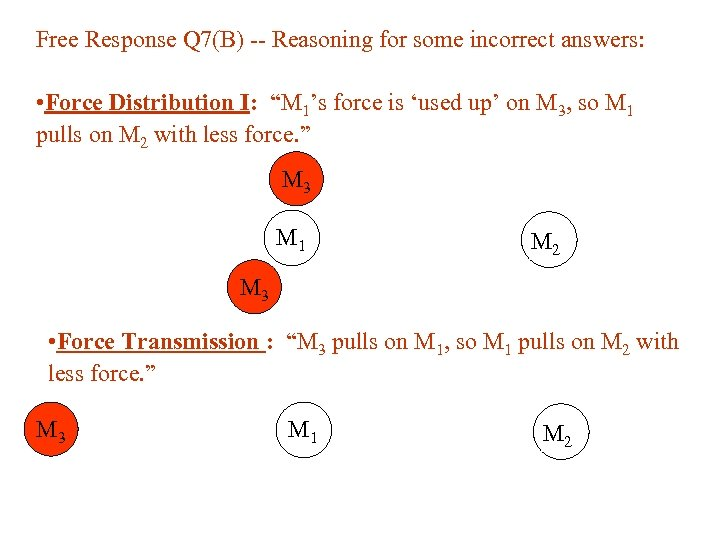Free Response Q 7(B) -- Reasoning for some incorrect answers: • Force Distribution I: “M 1’s force is ‘used up’ on M 3, so M 1 pulls on M 2 with less force. ” M 3 M 1 M 2 M 3 • Force Transmission : “M 3 pulls on M 1, so M 1 pulls on M 2 with less force. ” M 3 M 1 M 2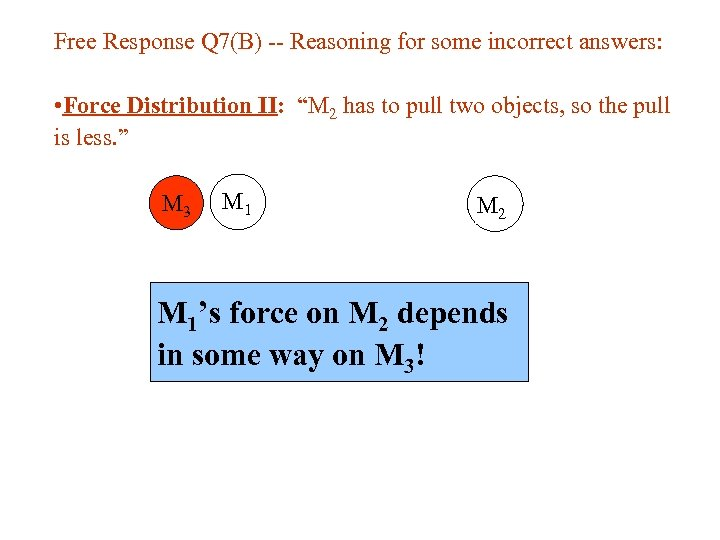Free Response Q 7(B) -- Reasoning for some incorrect answers: • Force Distribution II: “M 2 has to pull two objects, so the pull is less. ” M 3 M 1 M 2 M 1’s force on M 2 depends in some way on M 3!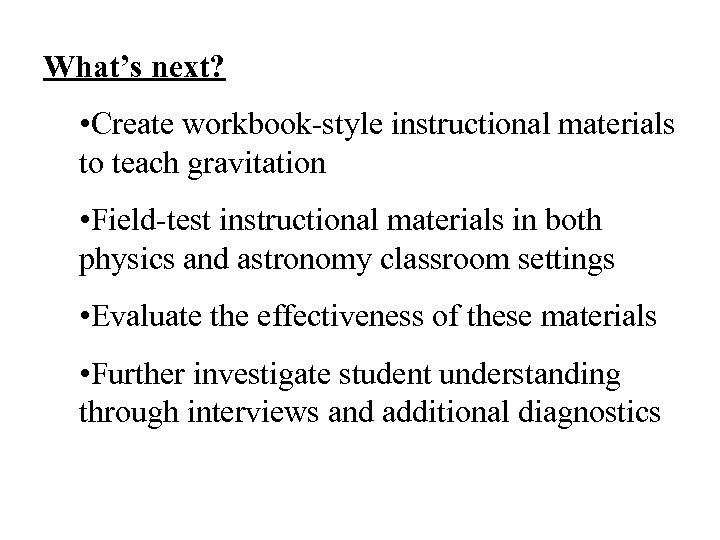What’s next? • Create workbook-style instructional materials to teach gravitation • Field-test instructional materials in both physics and astronomy classroom settings • Evaluate the effectiveness of these materials • Further investigate student understanding through interviews and additional diagnostics